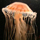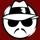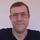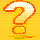## General Question# How can 1-2+3-4+5.... = 1/4 what isn't a integer?

Asked by dotlin (422) October 9th, 2010

Could you explain to me?

Observing members: 0Composing members: 0I give up. How?

FutureMemory (24721)“Great Answer” (0) Flag as…How familiar are you with the theory of

If you understand this you should type the following into the Wikipedia search box

1 − 2 + 3 − 4 + · · ·

because Fluther might not handle the URL

http://en.wikipedia.org/wiki/1_%E2%88%92_2_%2B_3_%E2%88%92_4_%2B_%C2%B7_%C2%B7_%C2%B7

properly. It should give you an article that starts like following:

“In mathematics, 1 − 2 + 3 − 4 + ··· is the infinite series whose terms are the successive positive integers, given alternating signs. Using sigma summation notation the sum of the first m terms of the series can be expressed as…

The infinite series diverges, meaning that its sequence of partial sums, (1, −1, 2, −2, …), does not tend towards any finite limit. Nonetheless, in the mid-18th century, Leonhard Euler wrote what he admitted to be a paradoxical equation:

A rigorous explanation of this equation would not arrive until much later. Starting in 1890, Ernesto Cesàro, Émile Borel and others investigated well-defined methods to assign generalized sums to divergent series—including new interpretations of Euler’s attempts. Many of these summability methods easily assign to 1 − 2 + 3 − 4 + … a “sum” of 1⁄4 after all. Cesàro summation is one of the few methods that does not sum 1 − 2 + 3 − 4 + …, so the series is an example where a slightly stronger method, such as Abel summation, is required.

The series 1 − 2 + 3 − 4 + … is closely related to Grandi’s series 1 − 1 + 1 − 1 + …. Euler treated these two as special cases of 1 − 2n + 3n − 4n + … for arbitrary n, a line of research extending his work on the Basel problem and leading towards the functional equations of what we now know as the Dirichlet eta function and the Riemann zeta function.”

mattbrowne (31633)“Great Answer” (5) Flag as…The series diverges. So strictly speaking it doesn’t have any value, because the partial sums do not converge to any number.

Probably the easiest way to make sense of this equality is as follows:

Using a geometric series formula you can get:

1+ x + x^2 + ... = 1/(1-x)

Now differentiate this and you get:

1+2x+3x^2+4x^3+... = 1/(1-x)^2.

Replace x =-1 and you get 1–2+3–4+... = ¼

This doesn’t really mean the value of this sum is ¼ but rather it means we can find a differentiable function whose values are the same as the values of 1+2x+3x^2+... and its value at -1 is ¼.

BonusQuestion (1483)“Great Answer” (4) Flag as…is there a website such as http://www.khanacademy.org/ that will explains series to me in more detail?

dotlin (422)“Great Answer” (0) Flag as…or